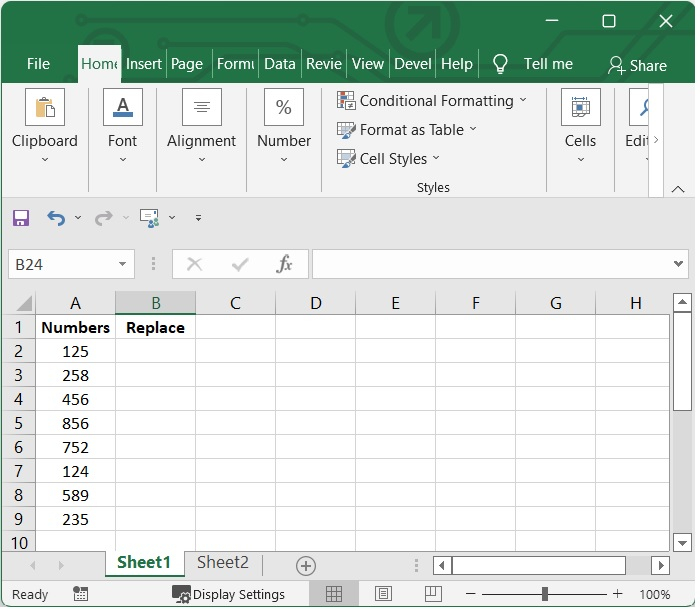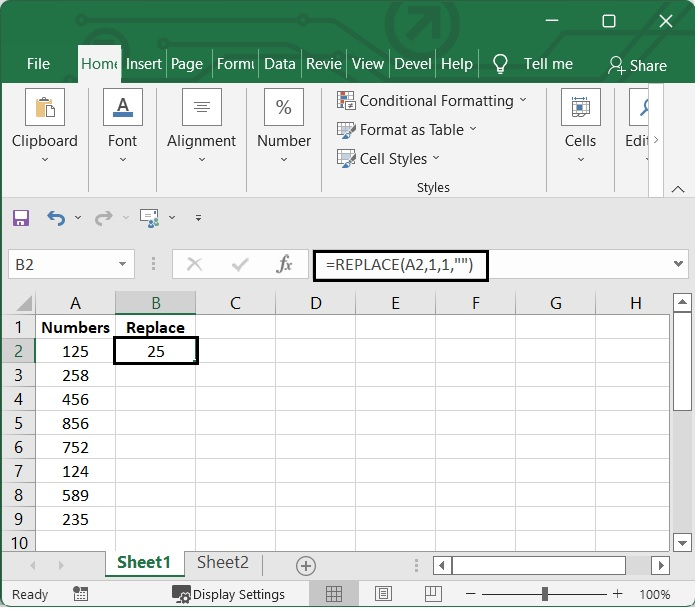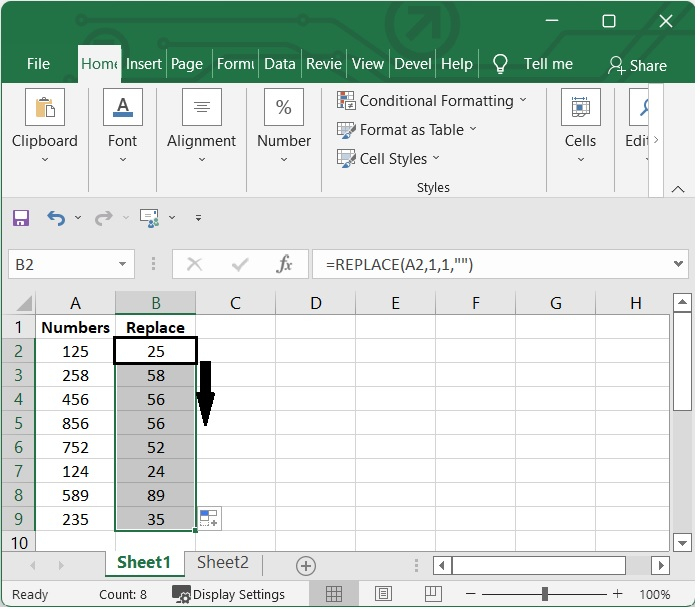# How to change or find and replace first number in cell in Excel?

You can search for something in your worksheet using the Find and Replace tools in Excel. Perhaps you're looking for a certain number or a text string to replace. You have the option of locating the object that was searched for as a point of reference or substituting it with something else.

In the search terms that you provide, you have the option of include numbers or wildcard characters like question marks, tildes, and asterisks. You are able to search by rows and columns, search within comments or values, search within worksheets or complete workbooks, and search within comments or values.

In this tutorial, we are going to show the way to replace the first number in cell.

## Using the Replace Function

When working with a large number of formulas, you may find that you need to modify a cell reference in each and every formula at some point. If you alter the value manually in each cell that contains a formula, it could take you a significant amount of time.

Excel's Find and Replace feature will come in useful at this point. It is able to quickly detect a cell reference anywhere in the worksheet's formulas or in the cells that have been selected and replace it with a different cell reference.

### Step 1

In our example, we have some numbers in excel sheet as shown below given image. We will remove first number in the cell by using Replace function.### Step 2

Then select one blank cell and add the below given formula in it. In our example we have selected the cell B2 and added the below given formula.

=REPLACE(A2,1,1,""


In our example we have applied the formula to the cell A2. You can change the cell as per your need.

The first number 1, which appears in the formula, denotes that the removal of the first number, and the second number 1, which appears in the formula, denotes that it will remove just one character from the left side of the cell.

After adding the formula press enter to see the result. See the below given formula.### Step 3

Select B2 cell and then to fill in the range using this formula, drag the Fill Handle to the appropriate location. Then you will notice that all of the first numbers in the cells that were referenced have been immediately removed.## Conclusion

In this tutorial, we explained how you can find and replace the first number in a cell in Excel.

Updated on: 06-Feb-2023

564 Views